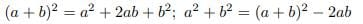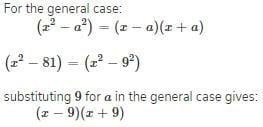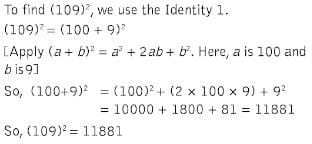Test Description

## 20 Questions MCQ Test Mathematics (Maths) Class 9 | Test: Algebraic Identities Quadratic

Test: Algebraic Identities Quadratic for Class 9 2023 is part of Mathematics (Maths) Class 9 preparation. The Test: Algebraic Identities Quadratic questions and answers have been prepared according to the Class 9 exam syllabus.The Test: Algebraic Identities Quadratic MCQs are made for Class 9 2023 Exam. Find important definitions, questions, notes, meanings, examples, exercises, MCQs and online tests for Test: Algebraic Identities Quadratic below.
Solutions of Test: Algebraic Identities Quadratic questions in English are available as part of our Mathematics (Maths) Class 9 for Class 9 & Test: Algebraic Identities Quadratic solutions in Hindi for Mathematics (Maths) Class 9 course. Download more important topics, notes, lectures and mock test series for Class 9 Exam by signing up for free. Attempt Test: Algebraic Identities Quadratic | 20 questions in 20 minutes | Mock test for Class 9 preparation | Free important questions MCQ to study Mathematics (Maths) Class 9 for Class 9 Exam | Download free PDF with solutions
 1 Crore+ students have signed up on EduRev. Have you?
Test: Algebraic Identities Quadratic - Question 1

### What should be subtracted to x2+2x+2 to make it a perfect square?

Detailed Solution for Test: Algebraic Identities Quadratic - Question 1

By subtracting 1 from 2,you will get 1 that makes the whole square of (x+1)=1

Test: Algebraic Identities Quadratic - Question 2

### If a + b = 12 and a2 + b2 = 60, the value of a3 + b3 is:

Detailed Solution for Test: Algebraic Identities Quadratic - Question 2

The correct option is Option C.

(a + b)² = a² + b² + 2ab

(12)² = 60 + 2ab

ab = (144 - 60)/2

ab = 84/2

ab = 42

Now,

(a + b)³ = a³ + b³ + 3ab(a+b)

(12)³ = a³ + b³ + (3 × 42 × 12)

a³ + b³ = 1728 - 1512

Therefore, a³ + b³ = 216

Test: Algebraic Identities Quadratic - Question 3

### One of the factors of (16y2 – 1) + (1 -4y)2 is​

Test: Algebraic Identities Quadratic - Question 4

(101)2 is equal to

Detailed Solution for Test: Algebraic Identities Quadratic - Question 4

(100-1)²= (100)²+(1)²+2×100×1 10000+1+200 =10201

Test: Algebraic Identities Quadratic - Question 5

Find the value of (9a + 10b)2

Test: Algebraic Identities Quadratic - Question 6

a2 + b2 + c2 – ab – bc – ca equals:

Detailed Solution for Test: Algebraic Identities Quadratic - Question 6

= ( a² + b² + c² - ab - bc - ca )

By multiplying it by 2 and dividing it by 2.

= 2 ( a² + b² + c² - ab - bc - ca ) ÷ 2

= ( 2a² + 2b² + 2c² - 2ab - 2bc - 2ca ) ÷ 2

= ( a² + a² + b² + b² + c² + c² - 2ab - 2bc - 2ca ) ÷ 2

= ( a² + b² - 2ab + b² + c² - 2bc + a² + c² - 2ca ) ÷ 2

= [ ( a - b )² + ( b - c )² + ( a - c )² ] ÷ 2

Now , whatever is the value of  ( a - b ) , ( b - c ) and ( a - c ) but its square will be always positive, and 2 is also a positive number.

So, the sum of ( a - b )², ( b - c )² and ( a - c )² will be a positive number and if a positive number is divided by a positive number then the result is also a positive number.

So, it is a positive number,hence it can't be a negative number.

Test: Algebraic Identities Quadratic - Question 7

Evaluate 98 x 102.​

Detailed Solution for Test: Algebraic Identities Quadratic - Question 7

(100+2)×(100-2). [(a+b)(a-b)=a^2-b^2
100^2 - 2^2
10000 - 4
9996

Test: Algebraic Identities Quadratic - Question 8

a2 + b2 is equal to​

Detailed Solution for Test: Algebraic Identities Quadratic - Question 8Test: Algebraic Identities Quadratic - Question 9

Solve the equation x2 + 6x - 7 = 0

Test: Algebraic Identities Quadratic - Question 10

If a - b = 3 and a2 + b2 = 29, find the value of ab.

Detailed Solution for Test: Algebraic Identities Quadratic - Question 10

2ab = (a2 + b2) - (a - b)2
= 29 - 9 = 20
⇒ ab = 10.

Test: Algebraic Identities Quadratic - Question 11

What should be added to x2+2x+0.5 to make it a perfect square?​

Detailed Solution for Test: Algebraic Identities Quadratic - Question 11

Let p(x) = x2 + 2x + 0.5

To find what must be added to p(x) to make it perfect square.

if we add 0.5 to p(x) we will get
p(x) = x2 + 2x + 0.5 + 0.5
p(x) = x2 + 2x + 1
p(x) = x2 + 1x + 1x + 1
p(x) = x(x + 1) + 1(x + 1)
p(x) = (x+1)(x+1)
p(x) = (x+1)2
which is a perfect square.

Therefore 0.5 must be added to the given expression to make it perfect square.

Test: Algebraic Identities Quadratic - Question 12

The missing figure in the expression
(5p +…)2 = ….+ 40 pq + 16q2 are:​

Test: Algebraic Identities Quadratic - Question 13

Factorise: x4-1

Test: Algebraic Identities Quadratic - Question 14

Factorise: x2– 81​

Detailed Solution for Test: Algebraic Identities Quadratic - Question 14Test: Algebraic Identities Quadratic - Question 15

What is the product of (x+a) and (x+b)?

Test: Algebraic Identities Quadratic - Question 16

The value of 101 x 99 is

Detailed Solution for Test: Algebraic Identities Quadratic - Question 16

(100+1)×(100 - 1) it is the formula of a²-b² so, (100)² -(1)² = 10000-1 = 9999.

Test: Algebraic Identities Quadratic - Question 17

If 2x + 3y = 12 and xy = 5, find the value of 4x2 + 9y2.

Test: Algebraic Identities Quadratic - Question 18

Find the value of (109)2

Detailed Solution for Test: Algebraic Identities Quadratic - Question 18Test: Algebraic Identities Quadratic - Question 19

(99)2 is equivalent to

Test: Algebraic Identities Quadratic - Question 20

The expansion of (2a – 3b + 5c)2.

Detailed Solution for Test: Algebraic Identities Quadratic - Question 20

The expansion of (2a - 3b + 5c)² -: (2a)² + (- 3b)² + (5c)² + 2× 2a × (-3b) + 2× (-3b)× 5c + 2× 5c + 2a. = 4a² + 9b² + 25c² - 12ab - 30bc + 20ac

## Mathematics (Maths) Class 9

88 videos|397 docs|109 tests
 Use Code STAYHOME200 and get INR 200 additional OFF Use Coupon Code